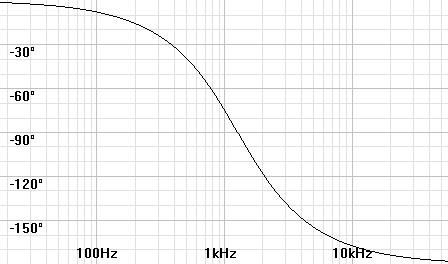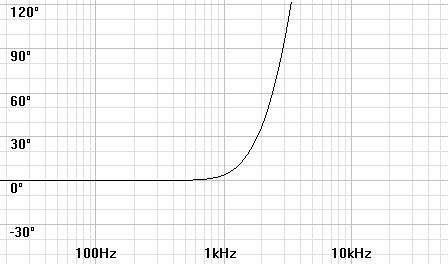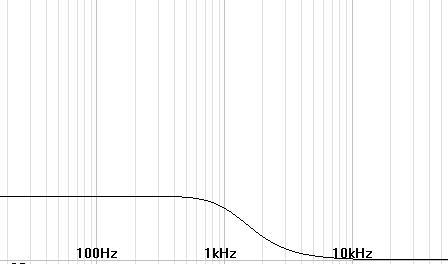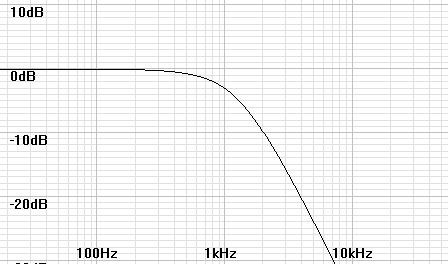Home  │ Audio Home Page

Updated January 3, 2009. See Document History at end for details.

# When Is Phase Shift not Phase Shift?

## Introduction

While contemplating things audio, it occurred to me that group delay was inconsistent with phase plots as far as lowpass responses go.  It appears that much of the phase shift of a typical phase shift plot is due to constant delay in the passband.  Indeed a fixed delay plotted as a phase shift graph with respect to input phase would be a straight diagonal line drawn from an origin of 0°/0Hz.  This is why lowpass responses that follow such a straight line through most of all their passband are called linear phase filters.  All this results from referring phase to input timing rather than the delayed timing of the majority of the passband.

## Solution

Compensate the phase calculation with a factor proportional to the frequency and passband group delay:

phasetrue = phasenormal + (delaygroup x frequency x 360°)

Here delaygroup represents the constant delay where where the group delay curve is flat.

## Application

Consider the following phase plots for a 1kHz 2nd Order Bessel Lowpass Filter, a linear phase filter that seems to have dismal phase shift according to a normal phase plot:

 Normal plot shows incorrect -74.33° phase shift at 1kHz Corrected plot shows correct +3.69° phase shift at 1kHzPassband group delay of  216.7µS is the basis for calculations. A frequency plot for completeness.## Disclaimer

If the normal phase plot above was plotted with a linear frequency scale rather than logarithmic you would see the phase follow a nearly straight line through the passband.

All group delay plots have delay in the band below the cutoff frequency regardless of type.  Therefore, this compensation does not apply to highpass responses because their group delay is in the stopband rather than the passband.  As for a bandpass filter, I expect ambiguous results because its passband falls in the transition region of the group delay plot.

Document History
January 2, 2009  created
January 3, 2009  revised